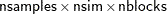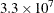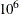### Computational Resources

It is impossible to estimate how long it will take for a general Markov chain to converge to its stationary distribution. It takes a skilled and thoughtful analysis of the chain to decide whether it has converged to the target distribution and whether the chain is mixing rapidly enough. In some cases, you might be able to estimate how long a particular simulation might take. The running time of a program that does not have RANDOM statements is approximately linear to the following factors: the number of samples in the input data set, the number of simulations, the number of blocks in the program, and the speed of your computer. For an analysis that uses a data set of size nsamples, a simulation length of nsim, and a block design of nblocks, PROC MCMC evaluates the log-likelihood function the following number of times, excluding the tuning phase:The faster your computer evaluates a single log-likelihood function, the faster this program runs. Suppose you have nsamples equal to 200, nsim equal to 55,000, and nblocks equal to 3. PROC MCMC evaluates the log-likelihood function approximatelytimes. If your computer can evaluate the log likelihood for one observationtimes per second, this program takes approximately a half a minute to run. If you want to increase the number of simulations five-fold, the run time increases approximately five-fold.

Each RANDOM statement adds one pass through the input data at each iteration. If the Metropolis algorithm is used to sample the random-effects parameter, the conditional density (objective function) is calculated twice per pass through the data, which requires a computational resource that is approximately equivalent to adding two blocks of parameters.

Of course, larger problems take longer than shorter ones, and if your model is amenable to frequentist treatment, then one of the other SAS procedures might be more suitable. With regular likelihoods and a lot of data, the results of standard frequentist analysis are often asymptotically equivalent to a Bayesian approach. If PROC MCMC requires too much CPU time, then perhaps another SAS/STAT tool would be suitable.